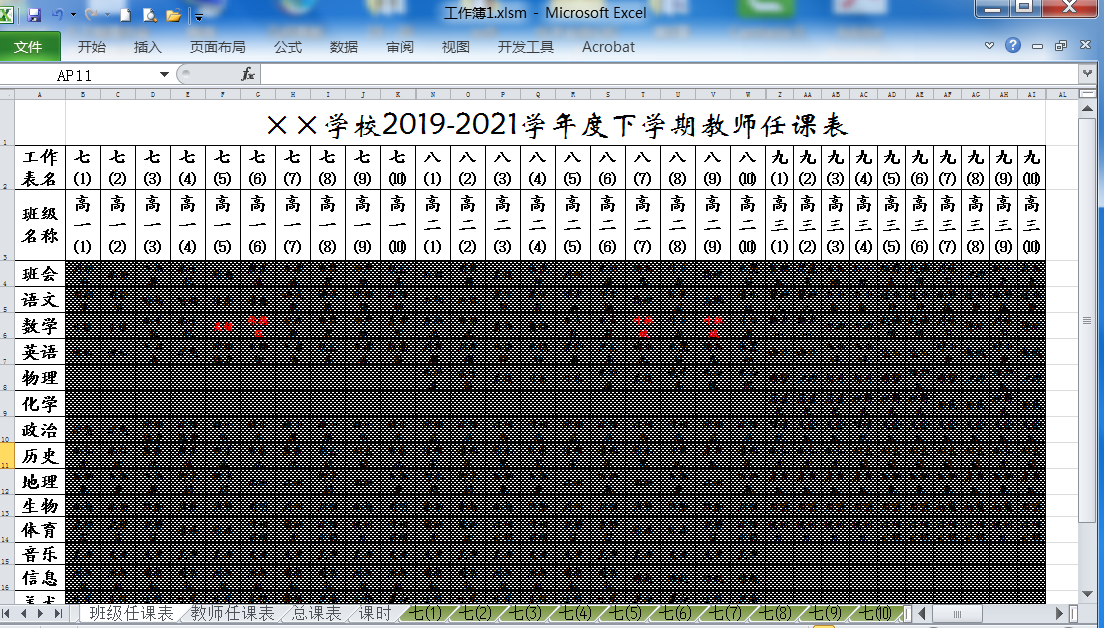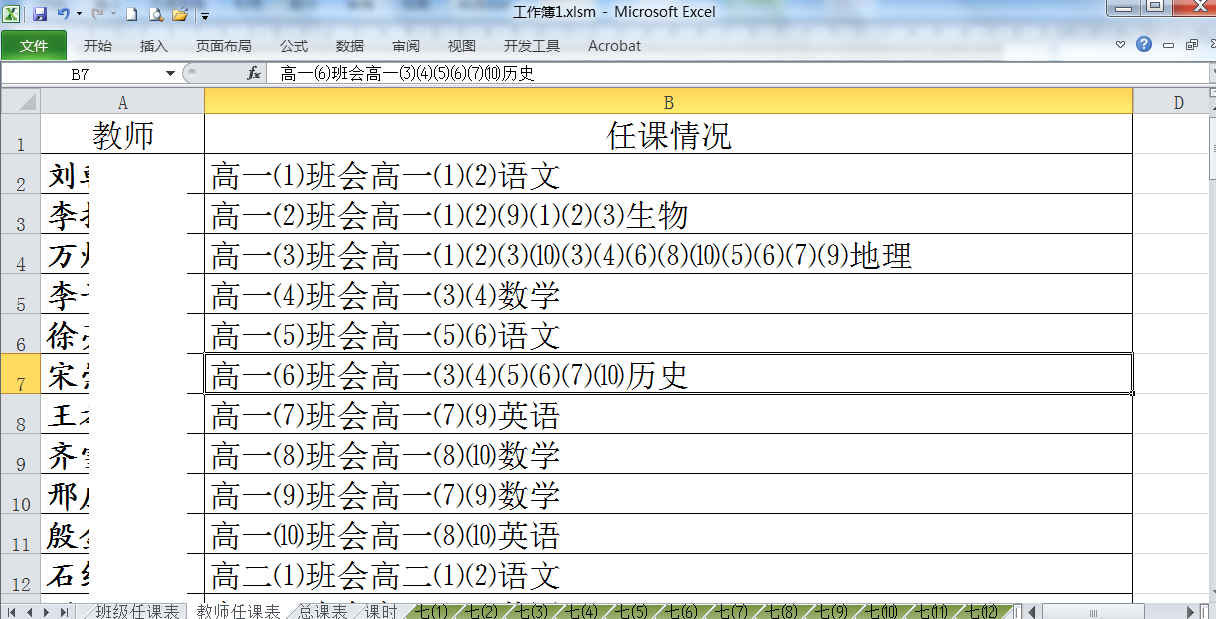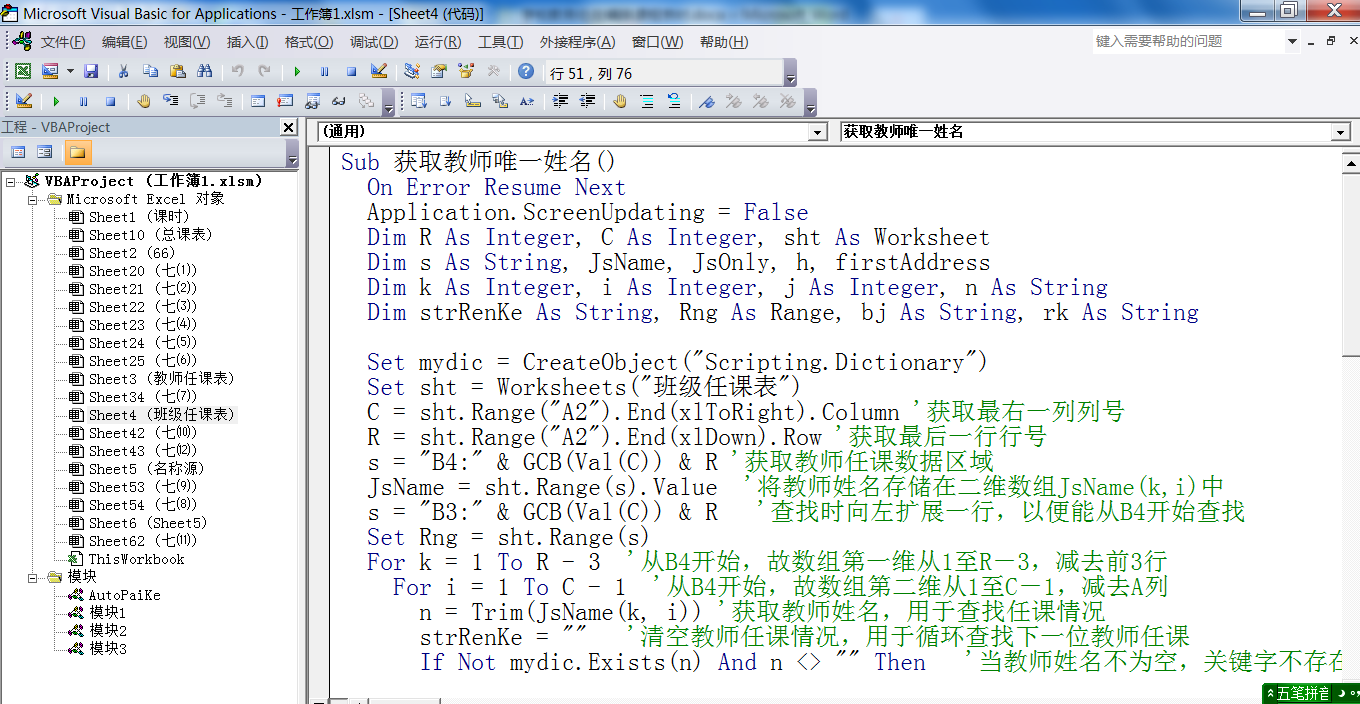一、问题的提出二、问题解决

1、《班级任课表》工作表的标签，从显示的快捷菜单中选择“显示代码”命令，在打开的VBA窗口中输入以下代码。

Sub获取教师的唯一名称() )

dim r as集成器、c as集成器、作为工作表

dim s as字符串，js名称，仅js，h，第一个地址

dim kas集成器，I as集成器，j as集成器，n as字符串

dim strr字符串、RNG as范围、bj as字符串、rk as字符串

setmydic=createobject (脚本.目录) )。

Set sht=Worksheets ('年级班主任表') ) )。

c=sht.range(a2) ).end ) xltoright ).Column )获取最右侧的列号

r=sht.range(a2) ).end ) XLdown ).Row )将获得最后一个行号

s='b4:'gcb(val ) c ) ) r

'获取教师的课程数据区域。 GCB是用于从列编号中获取列标签的自定义函数

s='b:'gcb(val(c ) ) r )向左扩展一行，以便在寻道时可以从B4进行寻道

setRNG=sht.range(s ) () ) ) ) ) ) ) ) ) ) )。

n=Trim(jsname(k，I ) ) )为了检索任意的授课状况，取得教师名

strRenKe=“”用于清除教师的授课情况，循环地寻找下一位教师的授课

ifnotmyDIC.exists(n ) And n '' Then '教师名称不为空，且关键字不存在

With Rng '搜索教师区域

bj='': rk='

seth=.find(n ) )。

If Not h Is Nothing Then

o

if左(BJ，1 )=左)右上.细胞) 3，h .列)，1 )安德拉克=右上，' a ' )三合一

BJ=SHT.Cells(3，h.Column ) '获取类名

strr enke=左(strr enke，len (strr enke-len ) rk ) )右)右，1 )左

ElseifRK=sht.cells(h.row，' a ' ) Then

BJ=sht.cells(3，h.Co

lumn) '获取班级名称

rk = sht.Cells(h.Row, "A") '获取学科名称

strRenKe = Left(strRenKe, Len(strRenKe) - Len(rk)) & bj & rk

Else

bj = sht.Cells(3, h.Column) '获取班级名称

rk = sht.Cells(h.Row, "A") '获取学科名称

strRenKe = strRenKe & bj & rk

End If

Set h = .FindNext(h)

End If

End With

End If

Next i

Next k

Set sht = Worksheets("教师任课表")

JsOnly = mydic.Keys '获取教师姓名，转置在A列

sht.Range("A2:A" & mydic.Count + 1) = WorksheetFunction.Transpose(JsOnly)

JsOnly = mydic.Items '获取教师任课，转置在B列

sht.Range("B2:B" & mydic.Count + 1) = WorksheetFunction.Transpose(JsOnly)

Set Rng = Nothing: Set sht = Nothing

Application.ScreenUpdating = True

End Sub

2、代码注释

⑴s = "B4:" & GCB(Val(C)) & R

C是获取最右一列列号，这与学校班级数量多少有关，这个C获取的是字符型数字，所以用Val函数才能将字符型数字C，转换成数值型数字。然后利用自定义函数GCB从列号获取列标字母，如37就转换为AK，从而获取教师任课数据区域。

⑵自定义函数GCB代码（这段代码最好放在模块中）

Public Function GCB(Num As Integer) As String

'快速将列标数字转化为列标（如2转化为B，16384转化为XFD等）

Application.ScreenUpdating = False

On Error Resume Next

If Num > 16384 Or Num < 1 Then Exit Function

Dim y As Integer, s As String

s = ""

Do

y = Num Mod 26

If y = 0 Then

s = Chr(64 + 26) & s

Num = Num \ 26 - 1

Else

s = Chr(64 + y) & s

Num = Num \ 26

End If

Loop Until Num = 0

GCB = Trim(s)

Application.ScreenUpdating = True

End Function

⑶Set mydic = CreateObject("Scripting.Dictionary")

⑷Set h = .Find(n)与Set h = .FindNext(h)

n储存的是一位教师的姓名，根据教师的姓名获取他的所有任课，Find是查找，FindNext是查找下一个直到查找完毕。

⑸Do……Loop循环中的多个If语句，用于处理教师任课中的冗余字符，譬如同一年级名称、同一学科名称只显示一个，这样数据更简洁直观。

⑹WorksheetFunction.Transpose(JsOnly)

JsOnly是储存教师姓名或教师任课的一维数组，对应于工作表中一行数据，要把一行数据转置为一列数据，使用工作表函数之转置函数WorksheetFunction.Transpose。VBA代码窗口

发表评论◎欢迎参与讨论，请在这里发表您的看法和观点。